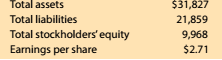Chapter 8, Problem 8.9.7MBA

Chapter
Section
Textbook Problem

Debt and price-earnings ratios Lowe's Companies Inc. (LOW) operates over 1,800 home improvement retail stores and is a competitor of The Home Depot (HD). The following data (in millions) were adapted from a recent financial statement of Lowe's:Compare the price-earninys ratios of Lowe's and The flotne Depot (MBA 8-8). Comment on any differences.

To determine

Concept Introduction:

Price Earnings Ratio:

The price earnings ratio shows the relationship between price of the share and earnings per share. It is calculated with the help of following formula:

Price Earnings Ratio=Market price per shareEarnings per share

To Indicate:

The Comment on the Price Earnings ratios

Explanation

The Price Earnings ratio for Company A is calculated as follows:

Price Earnings Ratio=Market price per shareEarnings per share

= 104.43/4.74

=22

Still sussing out bartleby?

Check out a sample textbook solution.

See a sample solution

The Solution to Your Study Problems

Bartleby provides explanations to thousands of textbook problems written by our experts, many with advanced degrees!

Get Started

Find more solutions based on key concepts